## Example Questions

### Example Question #1 : How To Find The Length Of The Side Of A Rectangle

The two rectangles shown below are similar. What is the length of EF?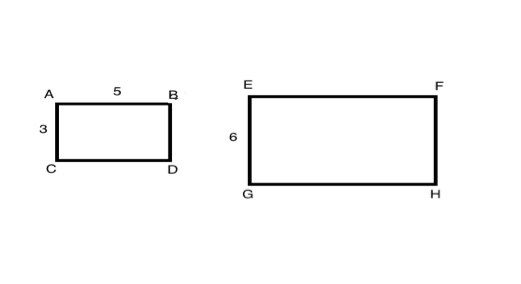Possible Answers:

6

10

8

5

Correct answer:

10

Explanation:

When two polygons are similar, the lengths of their corresponding sides are proportional to each other.  In this diagram, AC and EG are corresponding sides and AB and EF are corresponding sides.

To solve this question, you can therefore write a proportion:

AC/EG = AB/EF ≥ 3/6 = 5/EF

From this proportion, we know that side EF is equal to 10.

### Example Question #2 : How To Find The Length Of The Side Of A Rectangle

A rectangle is x inches long and 3x inches wide.  If the area of the rectangle is 108, what is the value of x?

Possible Answers:

3

6

8

4

12

Correct answer:

6

Explanation:

Solve for x

Area of a rectangle A = lw = x(3x) = 3x2 = 108

x2 = 36

x = 6

### Example Question #3 : How To Find The Length Of The Side Of A Rectangle

If the area of rectangle is 52 meters squared and the perimeter of the same rectangle is 34 meters. What is the length of the larger side of the rectangle if the sides are integers?

Possible Answers:

16 meters

14 meters

13 meters

15 meters

12 meters

Correct answer:

13 meters

Explanation:

Area of a rectangle is = lw

Perimeter = 2(l+w)

We are given 34 = 2(l+w) or 17 = (l+w)

possible combinations of l + w

are 1+16, 2+15, 3+14, 4+13... ect

We are also given the area of the rectangle is 52 meters squared.

Do any of the above combinations when multiplied together= 52 meters squared? yes 4x13 = 52

Therefore the longest side of the rectangle is 13 meters

### Example Question #1 : How To Find The Length Of The Side Of A Rectangle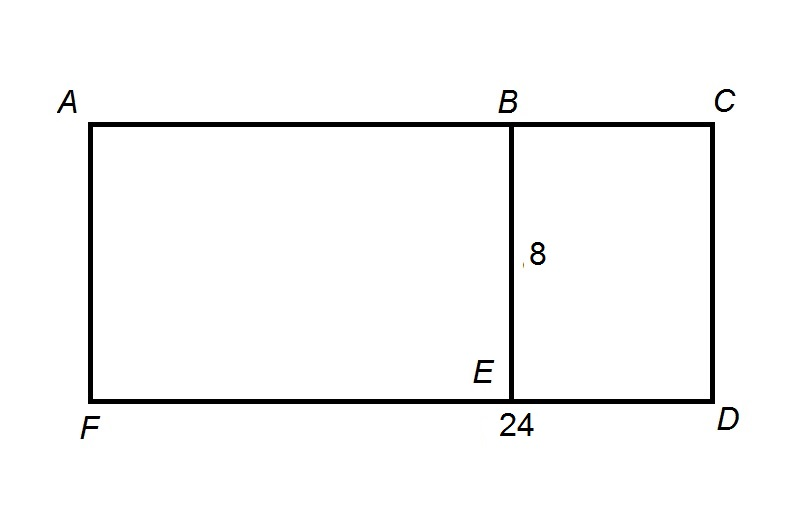Figure is not drawn to scale.

The provided figure is a rectangle divided into two smaller rectangles, with

Rectangle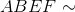Rectangle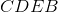.

Which expression is equal to the length of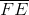?

Possible Answers: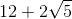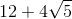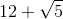Correct answer:Explanation:

Since Rectangle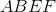is similar to Rectangle, it follows that corresponding sides are in proportion. Specifically,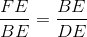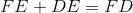;

since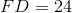, if we let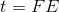, then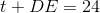,

and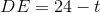Setting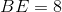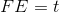, and, the proportion statement becomes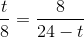Cross-multiplying, we get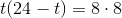Simplifying, we get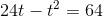Since this is quadratic, all terms must be moved to one side: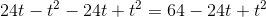or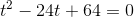The solutions to quadratic equation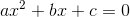can be found by way of the quadratic formula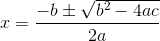Set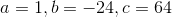: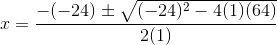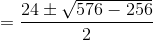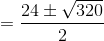Simplifying the radical using the Product of Radicals Property, we get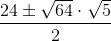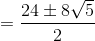Splitting the fraction and reducing: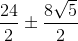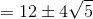This actually tells us that the lengths of the two segmentsand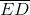have the lengthsand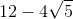is seen in the diagram to be the longer, so we choose the greater value,.

### All SAT Math Resources